# Harmonic space

(diff) ← Older revision | Latest revision (diff) | Newer revision → (diff)

A topological spacewith a (linear) sheafof continuous real-valued functions in which three fundamental properties of classical harmonic functions (cf. Harmonic function) are axiomatically fixed. These are: convergence as expressed by the second Harnack theorem; the maximum/minimum principle; and the solvability of the Dirichlet problem for a sufficiently broad class of open sets in. The functions inare called harmonic functions. The advantage of this axiomatic approach consists in the fact that if it is adopted, the theory comprises not only solutions of the Laplace equation, but also of certain other equations of elliptic and parabolic type. Letbe a locally compact topological space. A sheaf of functions onis understood to be a mapping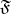defined on the family of all open sets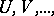ofsuch that: 1)is a family of functions on; 2) if, then the restriction of any function in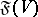tobelongs to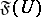; and 3) for any family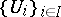, a function on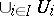belongs toif for allits restriction tobelongs to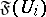. A sheaf of functionsis called hyperharmonic if, for any,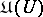is a convex cone of lower semi-continuous finite numerical functions on. A sheaf of functions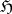is said to be harmonic if, for any,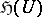is a real vector space of continuous functions on; the harmonic sheafis used in what follows.

A locally compact spaceis called a harmonic space if the following axioms are satisfied .

The positivity axiom:is non-degenerate at all points, i.e. for anythere exists a function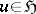defined in a neighbourhood ofsuch that.

The convergence axiom: If an increasing sequence of functions inis locally bounded, then it converges towards a function in.

The resolutivity axiom: There exists a basis of resolutive open sets, i.e. sets such that for any continuous functionof compact support on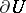there exists a solution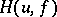of the Dirichlet problem forfrom, understood in the generalized sense of Wiener–Perron (cf. Perron method).

The axiom of completeness: If a lower semi-continuous function ofon, which is lower finite, satisfies the conditionon, for any relatively compact setwith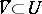, then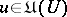.

The Euclidean space,, with the sheaf of classical solutions of the Laplace equation or of the thermal-conductance equation (heat equation) forms a harmonic space. There are several other variants of the axiomatics of harmonic spaces. Harmonic spaces are locally connected and do not contain isolated points; they have a basis of connected resolutive sets.

A hyperharmonic functionon a harmonic spaceis called superharmonic if for any relatively compact resolutive setthe function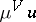is harmonic on. A positive superharmonic function for which any positive harmonic minorant is identically equal to zero is called a potential. A harmonic spaceis called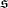-harmonic (-harmonic) if for anythere exists a positive superharmonic function(or, respectively, a potential) onsuch that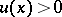.

Any harmonic space can be covered by open setswhich satisfy the minimum principle in the following form: If a hyperharmonic functionis positive outside the intersection ofwith any compact set inand if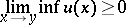for all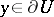, then. In the case of a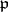-harmonic space this minimum principle is satisfied for all open sets. The Euclidean spacewith the sheaf of classical solutions of the Laplace equation is an-harmonic space if, and is a-harmonic space if and only if; the space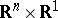,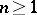, with the sheaf of solutions of the heat equation is a-harmonic space.

The principal problems of the theory of harmonic spaces include the theory of solvability of the Dirichlet problem, including the behaviour of the generalized solution of this problem at boundary points. The theory of the capacity of a set in a harmonic space, problems of balayage (cf. Balayage method) and the Robin problem have been studied.

How to Cite This Entry:
Harmonic space. E.D. Solomentsev (originator), Encyclopedia of Mathematics. URL: http://encyclopediaofmath.org/index.php?title=Harmonic_space&oldid=17695
This text originally appeared in Encyclopedia of Mathematics - ISBN 1402006098